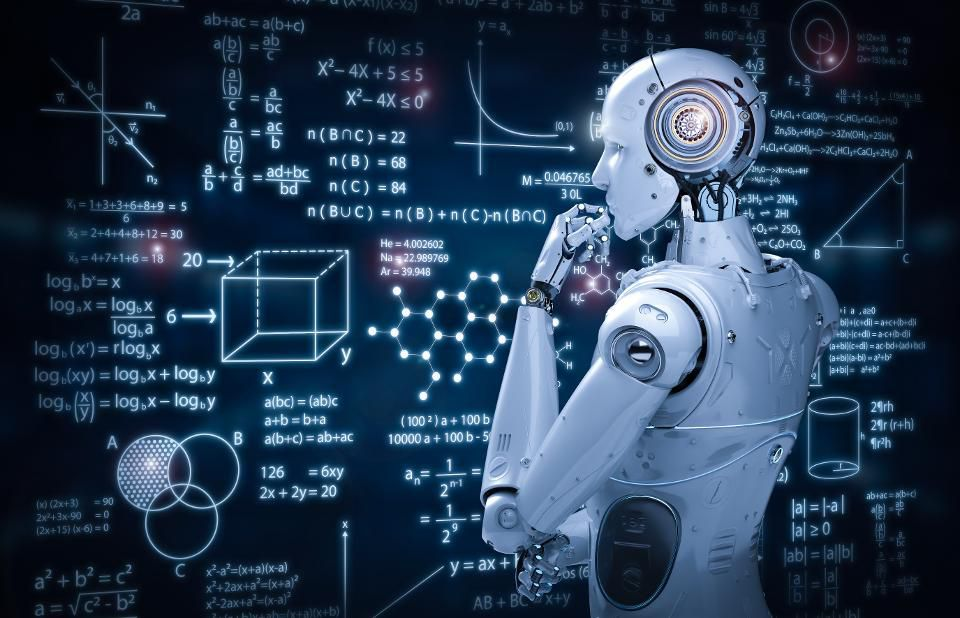Mon. Dec 16th, 2019

Lets Explore Machine Learning (Hamariweb)

# 5 Skills You Need to Become a Machine Learning Engineer

Machine LearningMachine Learning

Computer Science Fundamentals and Programming

Computer science fundamentals important for Machine Learning engineers include data structures (stacks, queues, multi-dimensional arrays, trees, graphs, etc.), algorithms (searching, sorting, optimization, dynamic programming, etc.), computability and complexity (P vs. NP, NP-complete problems, big-O notation, approximate algorithms, etc.), and computer architecture (memory, cache, bandwidth, deadlocks, distributed processing, etc.). You must be able to apply, implement, adapt or address them (as appropriate) when programming. Practice problems, coding competitions and hackathons are a great way to hone your skills.

2. Probability and Statistics

A formal characterization of probability (conditional probability, Bayes rule, likelihood, independence, etc.) and techniques derived from it (Bayes Nets, Markov Decision Processes, Hidden Markov Models, etc.) are at the heart of many Machine Learning algorithms; these are a means to deal with uncertainty in the real world. Closely related to this is the field of statistics, which provides various measures (mean, median, variance, etc.), distributions (uniform, normal, binomial, Poisson, etc.) and analysis methods (ANOVA, hypothesis testing, etc.) that are necessary for building and validating models from observed data. Many Machine Learning algorithms are essentially extensions of statistical modeling procedures.

3. Data Modeling and Evaluation

Data modeling is the process of estimating the underlying structure of a given dataset, with the goal of finding useful patterns (correlations, clusters, eigenvectors, etc.) and/or predicting properties of previously unseen instances (classification, regression, anomaly detection, etc.). A key part of this estimation process is continually evaluating how good a given model is. Depending on the task at hand, you will need to choose an appropriate accuracy/error measure (e.g. log-loss for classification, sum-of-squared-errors for regression, etc.) and an evaluation strategy (training-testing split, sequential vs. randomized cross-validation, etc.). Iterative learning algorithms often directly utilize resulting errors to tweak the model (e.g. backpropagation for neural networks), so understanding these measures is very important even for just applying standard algorithms.

4. Applying Machine Learning Algorithms and Libraries

Standard implementations of Machine Learning algorithms are widely available through libraries/packages/APIs (e.g. scikit-learn, Theano, Spark MLlib, H2O, TensorFlow etc.), but applying them effectively involves choosing a suitable model (decision tree, nearest neighbor, neural net, support vector machine, ensemble of multiple models, etc.), a learning procedure to fit the data (linear regression, gradient descent, genetic algorithms, bagging, boosting, and other model-specific methods), as well as understanding how hyperparameters affect learning. You also need to be aware of the relative advantages and disadvantages of different approaches, and the numerous gotchas that can trip you (bias and variance, overfitting and underfitting, missing data, data leakage, etc.). Data science and Machine Learning challenges such as those on Kaggle are a great way to get exposed to different kinds of problems and their nuances.

5. Software Engineering and System Design

At the end of the day, a Machine Learning engineer’s typical output or deliverable is software. And often it is a small component that fits into a larger ecosystem of products and services. You need to understand how these different pieces work together, communicate with them (using library calls, REST APIs, database queries, etc.) and build appropriate interfaces for your component that others will depend on. Careful system design may be necessary to avoid bottlenecks and let your algorithms scale well with increasing volumes of data. Software engineering best practices (including requirements analysis, system design, modularity, version control, testing, documentation, etc.) are invaluable for productivity, collaboration, quality and maintainability.

#### You may have missed2 min read

#### Formatting and Spacing Guidelines For Effective CV Writing4 min read

#### How to write a CV: 10 Top Tips for 20195 min read

#### Top 40 Electronics Interview Questions updated on Sep 2019 Part 24 min read